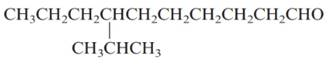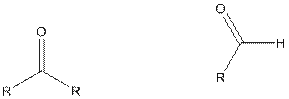Chapter 20.14, Problem 20.7SC### Introductory Chemistry: A Foundati...

9th Edition
Steven S. Zumdahl + 1 other
ISBN: 9781337399425

#### Solutions

Chapter
Section### Introductory Chemistry: A Foundati...

9th Edition
Steven S. Zumdahl + 1 other
ISBN: 9781337399425
Textbook Problem
1 views

# Exercise 20.7 Name the following molecules.Interpretation Introduction

(a)

Interpretation:

The name of the given molecule should be determined.

Concept Introduction:

Aldehyde and ketone both contains carbonyl group. In case of aldehyde, carbonyl group is present at the end of the hydrocarbon chain and in case of ketone, carbonyl group is present between two carbon atoms.

The general formula of ketone and aldehyde is:Where, R is an alkyl group. In case of ketone, R group can be same on both sides or can be different.

Now, the naming of aldehyde and ketone is given as:

1. First choose the longest continuous chain of carbon atoms having carbonyl group known as parent chain.
2. The numbering of parent chain should be done in a way that the carbonyl group and substituents get the lowest number.
3. The root name of the carbon chain is same as in case of alkanes, but “−e” ending is replaced by “−al” in case of aldehyde and in case of ketone, “−e” ending is replaced by “−one”.
4. The appropriate name should be given to every alkyl group (substituent) as and denote its position on the parent chain with the number.
5. The alkyl groups are written in alphabetical order.
Explanation

The given structure is

1. The longest continuous chain contains six carbon atoms thus, the prefix hexa is used.

2. One substituent that is ethyl group is present on fourth carbon at...

Interpretation Introduction

(b)

Interpretation:

The name of the given molecule should be determined.

Concept Introduction:

Aldehyde and ketone both contains carbonyl group. In case of aldehyde, carbonyl group is present at the end of the hydrocarbon chain and in case of ketone, carbonyl group is present between two carbon atoms.

The general formula of ketone and aldehyde is:Where, R is an alkyl group. In case of ketone, R group can be same on both sides or can be different.

Now, the naming of aldehyde and ketone is given as:

1. First choose the longest continuous chain of carbon atoms having carbonyl group known as parent chain.
2. The numbering of parent chain should be done in a way that the carbonyl group and substituents get the lowest number.
3. The root name of the carbon chain is same as in case of alkanes, but “−e” ending is replaced by “−al” in case of aldehyde and in case of ketone, “−e” ending is replaced by “−one”.
4. The appropriate name should be given to every alkyl group (substituent) as and denote its position on the parent chain with the number.
5. The alkyl groups are written in alphabetical order.

### Still sussing out bartleby?

Check out a sample textbook solution.

See a sample solution

#### The Solution to Your Study Problems

Bartleby provides explanations to thousands of textbook problems written by our experts, many with advanced degrees!

Get Started